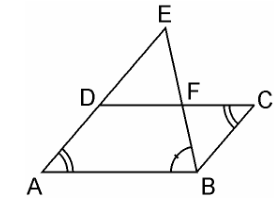# E is a point on the side AD produced of a parallelogram ABCD and BE intersects CD at F. Show that ΔABE ~ ΔCFB.

Given

E is a point on the side AD produced of a parallelogram ABCD and BE intersects CD at F.

To Prove

ΔABE ~ ΔCFB

ProofE is a point on the side AD produced of a parallelogram ABCD and BE intersects CD at F.

Now,

In ΔABE and ΔCFB,

Since, the opposite angles of a parallelogram are equal, we have,

∠A = ∠C

Also, since AE || BC

∠AEB = ∠CBF (Alternate interior angles)

By AA similarity criterion,

∴ ΔABE ~ ΔCFB

Hence Proved